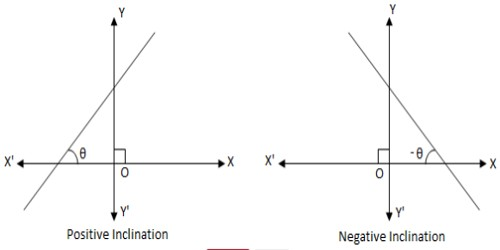Inclination of a Line

The inclination of line is the angle θ which the part of the line (above the x-axis) makes with x-axis. The angle between a line and the x-axis. This angle is always between 0° and 180°, and is measured counterclockwise from the part of the x-axis to the right of the line.

All horizontal lines have angle of inclination 0°. All vertical lines have angle of inclination 90°. Also, the slope of a line is given by the tangent of the angle of inclination.If measured in anti-clockwise direction then the inclination θ is positive and if measured in clockwise direction then the inclination θ is negative.

The inclination of a line or the angle of inclination of the line is the angle which a straight line makes with the positive direction of x-axis measured in the anti-clockwise direction to the part of the line above the x-axis. The inclination is usually denoted by θ.

Therefore, if the angle or the slope is known, the other can be found using one of the equations. If the angle of inclination is negative, then the slope of the line is also negative.

Remember:

(i) The inclination of x-axis and every line parallel to x-axis is 0°

i.e. the inclination of a line parallel to x-axis or the x-axis itself is 0°.

(ii) The inclination of y-axis and every line parallel to y-axis is 90°

i.e. the inclination of a line parallel to y-axis or the y-axis itself is 90°.

Information Source: